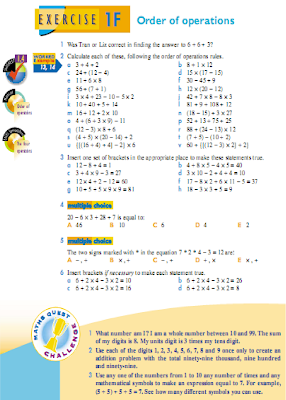## Kamis, 04 Agustus 2011

### ExerciseEXERCISE

1. Fill in the correct signs (< or >) in place of the * in each example to show which of these
heigts above and below sea level is the greather.
a. 150 m * 75 m b. 50 m * -75 m
c. -65 m * 30 m d. 0 m * -20 m
e. -10 m * -20 m f. -25 m * 25 m

2. Find the value of :
a. (+5) + (+3) b. (-4) + (+7)
c. (+6) + (-2) d. (-1) + (-2)
e. (+3) - (+8) f. (+2) - (-3)
g. (-5) - (+1) h.(-5) - (-6)

3. On a certain day at certain time in Siberia, the temperature was - 33 0 C. On the same day at
the same time , the temperatur in Brazil was 330 C. What is the difference between these two
temperetures ?.

4. A Submarine is 50 m below sea level.
a. It goes down a futher 280 m to point P. Write down the depth of the point P below sea
level .
b. From point P it rises 110 m to point Q . What the depth of Q below sea level ?

5. The temperatur on freezer thermometer shows that food is being stored at -20 o C.
a. What would the temperature be if was raised by 5 o C ?
b. What would the temperature be if it lowered by 0.5 o C every hour for 12 hours ?